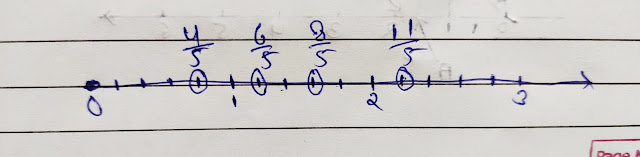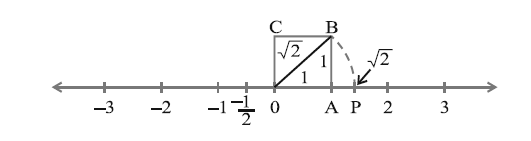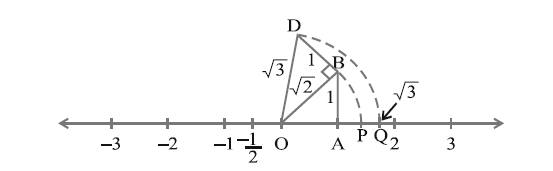# Number System- Rational & Irrational Number Numbers

## What is a Rational Number? Definition-

A number "r' is called a rational number if it can be written in the form of p/q, where p and q are integers and have no common factors(except 1), and also q is not equal to 0.
Now all the numbers in your can be written in the form p/q, where p and q are integers and, q is not equal to 0. there are infinitely rational numbers.
for example- 12 can be written as 12/1,  where p=12, and q=1. Therefore, The rational number also included the natural number, whole number, and integers.

#### Locating Rational numbers on Number line-

Locate These Rational numbers on Number line (see, what is number line? First)-
4/5, 6/5, 8/5, and 11/5Number Line

## What is Irrational number?

We saw, in the previous section, That the number which can be written in the form of P/q where q is not equal to 0 is a Rational number. But, there must be numbers in your mind that can't be written in the form of p/q. In this section, we are going to investigate those numbers.
A number 's' is called an Irrational number, if it can not be written in the form of p/q, where p and q are integers and q is not equal to 0.
we already know that there are infinitely many rational numbers. it turns out that there are infinitely irrationals too. Some examples are-
√2, √3, √15, π, etc.
Recall- recall that when we use the symbol √, we assume that it is the positive square root of the number. So, √4 = 2, Though both 2 and -2 are square roots of 4.Pythagoras
The Pythagoras proved that √2 is an Irrational number. later in approximately in 425BC, Theodores of Cyrene showed that √3, √5, √6, √7, √10, √11, √12, √13, √14, √15, and √17 are also irrationals.

#### Locating Irrational numbers on the number line-

Locate √2 on the number line-
Consider a unit square OABC, with each side 1 unit in length (see below fig.). now you can get √2 using Pythagoras theorem. So, according to  Pythagoras theorem, OA² + AB² = BO² So, BO = √(OA² + AB²),
So, √2 = √(1² + 1²)
So we have just find OB = √2. now, Draw an arc Using a compass with center O and Radius OB, which intersecting the number line at the Point P. The Point P corresponds to √2 on the number line.√2 on Number line

Locate √3 on the number line-
Line DO = √(OB² + BD²),
So, DO = √{(√2)² + 1²} = √3√3 on Number line